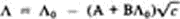# Onsager Equation of Electrical Conductivity

## Onsager Equation of Electrical Conductivity

a relationship that shows the dependence of the electrical conductivity of an electrolyte on its concentration. The equation is valid for highly dilute solutions of strong electrolytes. In the simplest case, for a fully dissociated mono-monovalent electrolyte (such as NaCl), the Onsager equation of electrical conductivity assumes the formHere Λ is the equivalent electrical conductivity, Λ0 is the equivalent electrical conductivity for infinite dilution, c is the concentration in moles per liter, andwhere ∊ is the dielectric constant, η is the viscosity in poises, and T is the absolute temperature (°K). The Onsager equation of electrical conductivity was derived by L. Onsager.

Site: Follow: Share:
Open / Close